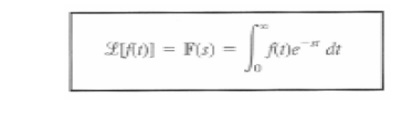Home | | Signals and Systems | Laplace Transform

# Laplace Transform

Lapalce transform is a generalization of the Fourier transform in the sense that it allows ÔÇťcomplex frequencyÔÇŁ whereas Fourier analysis can on ly handle ÔÇťreal frequencyÔÇŁ.

Laplace Transform

Lapalce transform is a generalization of the Fourier transform in the sense that it allows ÔÇťcomplex frequencyÔÇŁ whereas Fourier analysis can on ly handle ÔÇťreal frequencyÔÇŁ. Like Fourier transform, Lapalce transform allows us to analyze a ÔÇťlinear circuitÔÇŁ problem, no matter how complicated the circuit is, in the frequency domain in stead of in he time domain.

Mathematically, it produces the benefit of converting a set of differential equations into a corresponding set of algebraic equations, which are much easier to solve. Physically, it produces more insight of the circuit and allows us to know the bandwidth, phase, and transfer characteristics important for circuit analysis and design.

Most importantly, Laplace transform lifts the limit of Fourier analysis to allow us to find both the steady-state and ÔÇťtransientÔÇŁ responses of a linear circuit. Using Fourier transform, one can only deal with he steady state behavior (i.e. circuit response under indefinite sinusoidal excitation).

Using Laplace transform, one can find the response under any types of excitation (e.g. switching on and off at any given time(s), sinusoidal, impulse, square wave excitations, etc.Study Material, Lecturing Notes, Assignment, Reference, Wiki description explanation, brief detail
Signals and Systems : Analysis of Continuous Time Signals : Laplace Transform |